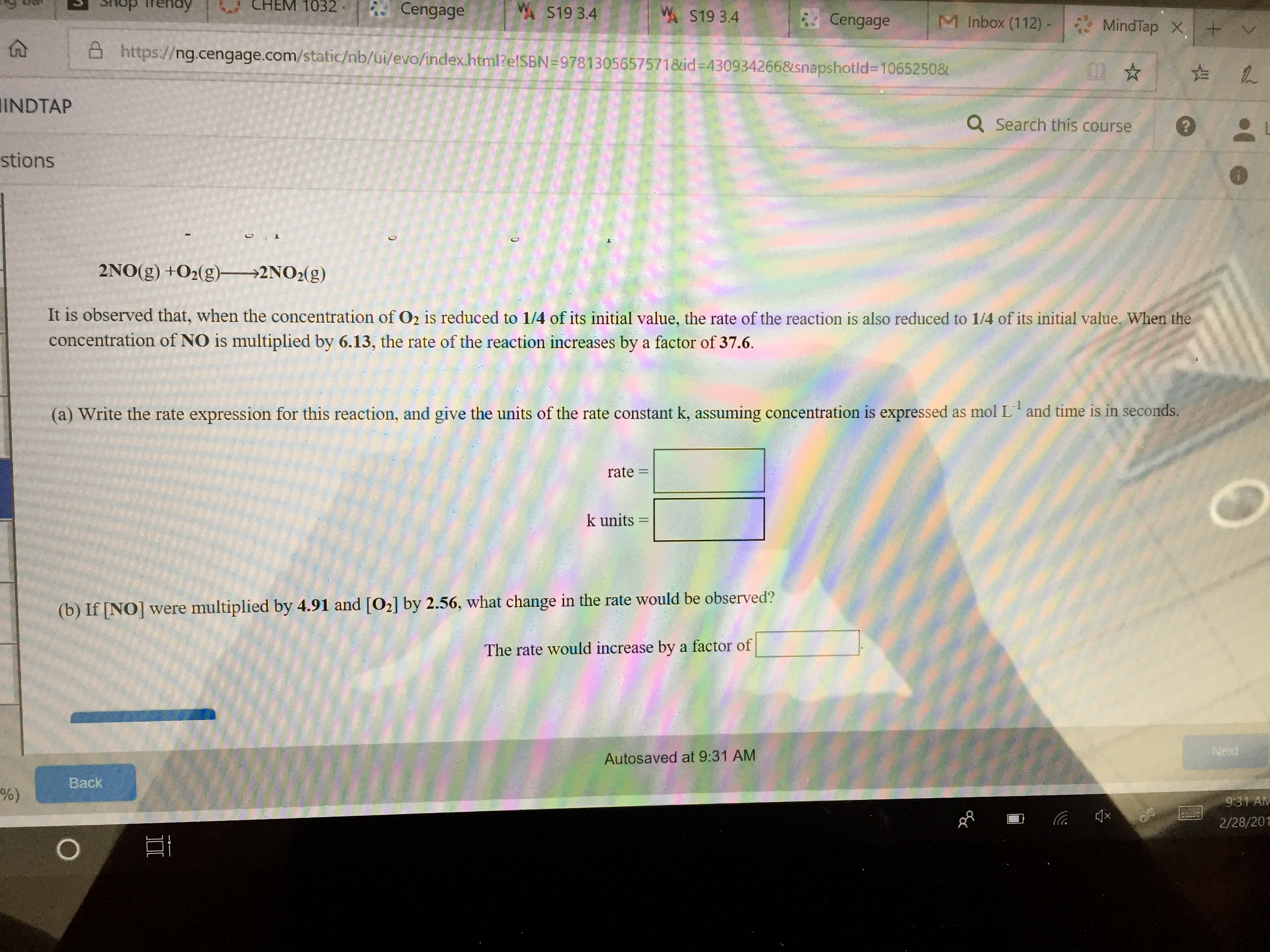# A S19 3.4 "Х S19 3.4 . Cengage | M Inbox (112)-| MindTap × I+ ttps:// ng.cengage.com/static/nb/ui/evo/index.html?e ISBN #9781 305 65757 1 &id-4309342 66&snapshot id-1 065250& INDTAP Q search this course stions 2NO(g) +02(g) 2NO2(g) It is observed that, when the concentration of O2 is reduced to 1/4 of its initial value, the rate of the reaction is also reduced to 1/4 of its initial value, When the concentration of NO is multiplied by 6.13, the rate of the reaction increases by a factor of 37.6. (a) Write the rate expression for this reaction, and give the units of the rate constant k, assuming concentration is expressed as mol L and time is in seconds rate = k units- (b) If INO] were multiplied by 4.91 and [O2] by 2.56, what change in the rate would be observed? The rate would increase by a factor of Next Autosaved at 9:31 AM Back 9:31 Alv 2/28/201

Questionhelp_outlineImage TranscriptioncloseA S19 3.4 "Х S19 3.4 . Cengage | M Inbox (112)-| MindTap × I+ ttps:// ng.cengage.com/static/nb/ui/evo/index.html?e ISBN #9781 305 65757 1 &id-4309342 66&snapshot id-1 065250& INDTAP Q search this course stions 2NO(g) +02(g) 2NO2(g) It is observed that, when the concentration of O2 is reduced to 1/4 of its initial value, the rate of the reaction is also reduced to 1/4 of its initial value, When the concentration of NO is multiplied by 6.13, the rate of the reaction increases by a factor of 37.6. (a) Write the rate expression for this reaction, and give the units of the rate constant k, assuming concentration is expressed as mol L and time is in seconds rate = k units- (b) If INO] were multiplied by 4.91 and [O2] by 2.56, what change in the rate would be observed? The rate would increase by a factor of Next Autosaved at 9:31 AM Back 9:31 Alv 2/28/201 fullscreen

### Want to see the step-by-step answer?